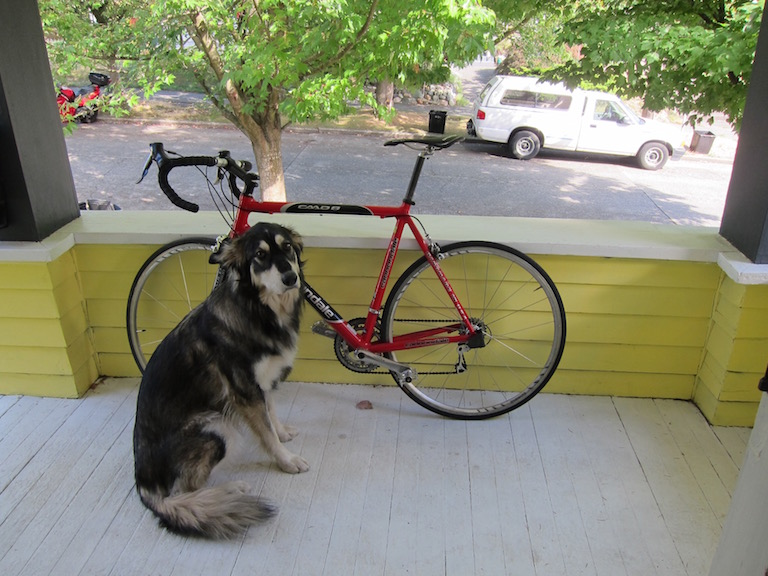# Object detection using a model zoo model

Object detection is a computer vision technique for locating instances of objects in images or videos.

In this example, you learn how to implement inference code with a ModelZoo model to detect dogs in an image.

The source code can be found at ObjectDetection.java.

You can also use the Jupyter notebook tutorial. The Jupyter notebook explains the key concepts in detail.

## Run object detection example

### Input image file

You can find the image used in this example in the project test resource folder: `src/test/resources/dog_bike_car.jpg`### Build the project and run

Use the following command to run the project:

``````cd examples
``````

Your output should look like the following:

``````[INFO ] - Detected objects image has been saved in: build/output/detected-dog_bike_car.png
[INFO ] - [
class: "car", probability: 0.99991, bounds: [x=0.611, y=0.137, width=0.293, height=0.160]
class: "bicycle", probability: 0.95385, bounds: [x=0.162, y=0.207, width=0.594, height=0.588]
class: "dog", probability: 0.93752, bounds: [x=0.168, y=0.350, width=0.274, height=0.593]
]
``````

An output image with bounding box will be saved as build/output/detected-dog_bike_car.png: